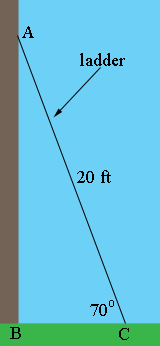SEARCH HOMEMath Central Quandaries & QueriesQuestion from Al, a student: a ladder is leaning against a wall at a 70 degree angle and the ladder is 20 feet tall...how far away is the ladder from the wallAl,

The very first step is to draw a diagram.At the top the ladder is touching the wall so I think you want to know how far the base of the ladder is from the wall, that is what is the distance from B to C?

Triangle ABC is a right triangle, the length of the hypotenuse is 20 ft and you want to know the length of the side adjacent to the 70o angle. What trig function relates the measure of an angle, the adjacent side and the hypotenuse?

PennyMath Central is supported by the University of Regina and The Pacific Institute for the Mathematical Sciences.